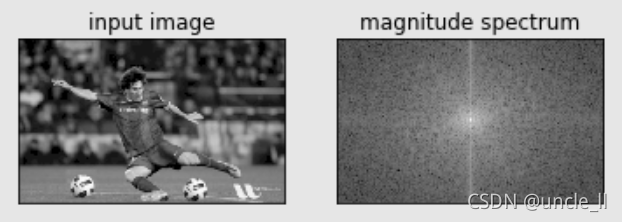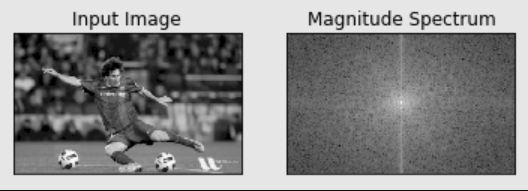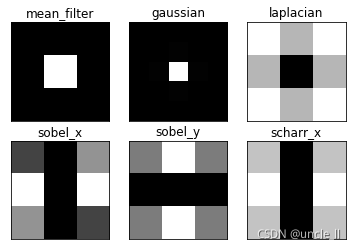# OpenCV 24: 傅立葉變換

### Numpy中的傅立葉變換

np.fft.fft2() * 第一個引數是輸入影象，即灰度影象。 * 第二個引數是可選的，決定輸出陣列的大小。 如果它大於輸入影象的大小，則在計算FFT之前用零填充輸入影象。如果小於輸入影象，將裁切輸入影象。如果未傳遞任何引數，則輸出陣列的大小將與輸入的大小相同。

img = cv2.imread('messi.png', 0) f = np.fft.fft2(img) fshift = np.fft.fftshift(f)

magnitude_spectrum = 20 * np.log(np.abs(fshift))

plt.subplot(121) plt.imshow(img, cmap='gray')

plt.title('input image') plt.xticks([]) plt.yticks([])

plt.subplot(122) plt.imshow(magnitude_spectrum, cmap='gray') plt.title('magnitude spectrum') plt.xticks([]) plt.yticks([])

plt.show()可以看到，在中心看到更多白色區域，這表明低頻內容更多

# ifft

import cv2 import numpy as np from matplotlib import pyplot as plt

img = cv2.imread('messi.png', 0) f = np.fft.fft2(img) fshift = np.fft.fftshift(f)

rows, cols = img.shape crow, ccol = rows//2, cols//2 fshift[crow-30: crow+31, ccol-30:ccol+31] = 0 f_ishift = np.fft.ifftshift(fshift) img_back = np.fft.ifft2(f_ishift) img_back = np.real(img_back) # 取實部

plt.subplot(131) plt.imshow(img, cmap = 'gray') plt.title('Input Image') plt.xticks([]) plt.yticks([]) plt.subplot(132) plt.imshow(img_back, cmap = 'gray') plt.title('Image after HPF') plt.xticks([]) plt.yticks([]) plt.subplot(133) plt.imshow(img_back, cmap='gray') plt.title('Result in JET') plt.xticks([]) plt.yticks([])

plt.show()### OpenCV中的傅立葉變換

OpenCV為此提供了cv2.dft()cv2.idft()函式。它返回與前一個相同的結果，但是有兩個通道。 * 第一個通道是結果的實部 * 第二個通道是結果的虛部。

python

# opencv

import cv2 import numpy as np from matplotlib import pyplot as plt

img = cv2.imread('messi.png', 0) img_32 = np.float32(img) dft = cv2.dft(img_32, flags=cv2.DFT_COMPLEX_OUTPUT) dft_shift = np.fft.fftshift(dft)

magnitude_spectrum = 20*np.log(cv2.magnitude(dft_shift[:, :, 0], dft_shift[:, :, 1]))

plt.subplot(121) plt.imshow(img, cmap = 'gray') plt.title('Input Image') plt.xticks([]) plt.yticks([]) plt.subplot(122) plt.imshow(magnitude_spectrum, cmap = 'gray') plt.title('Magnitude Spectrum') plt.xticks([]) plt.yticks([]) plt.show()rows, cols = img.shape crow, ccol = rows//2, cols//2 print(crow, ccol)

# apply mask and inverse DFT

fshift = dft_shift * mask f_ishift = np.fft.ifftshift(fshift)

img_back = cv2.idft(f_ishift) img_back = cv2.magnitude(img_back[:, :, 0], img_back[:, :, 1])

plt.subplot(121) plt.imshow(img, cmap = 'gray') plt.title('Input Image') plt.xticks([]) plt.yticks([]) plt.subplot(122) plt.imshow(img_back, cmap = 'gray') plt.title('Magnitude Spectrum') plt.xticks([]), plt.yticks([]) plt.show() 

### DFT的效能優化

python

# performance

img = cv2.imread('messi.png', 0) rows, cols = img.shape print("{}, {}".format(rows, cols))

# 259, 419

nrows = cv2.getOptimalDFTSize(rows) ncols = cv2.getOptimalDFTSize(cols) print("{}, {}".format(nrows, ncols))

# 270, 432

可以看到，將大小(259, 419)修改為(270，432)。現在用零填充（對於OpenCV），並找到其DFT計算效能。可以通過建立一個新的零陣列並將資料複製到其中來完成此操作，或者使用cv2.copyMakeBorder()。python nimg = np.zeros((nrows,ncols)) nimg[:rows,:cols] = img 

# 15.4 ms ± 1.22 ms per loop (mean ± std. dev. of 7 runs, 100 loops each)

%timeit fft2 = np.fft.fft2(img,[nrows,ncols])



# 3.21 ms ± 217 µs per loop (mean ± std. dev. of 7 runs, 100 loops each)

%timeit dft2= cv2.dft(np.float32(nimg),flags=cv2.DFT_COMPLEX_OUTPUT)



# laplacian is high pass filter

import cv2 import numpy as np from matplotlib import pyplot as plt

# simple averaging filter without scaling parameter

mean_filter = np.ones((3, 3))

# creating a gaussian filter

x = cv2.getGaussianKernel(5, 10) gaussian = x*x.T

# scharr in x-direction

scharr_x = np.array([[-3, 0, 3], [-10, 0, 10], [-3, 0, 3]])

# sobel in x direction

sobel_x = np.array([[-1, 0, -1], [-2, 0, 2], [-1, 0, 1]])

# sobel in y directio

sobel_y= np.array([[-1,-2,-1], [0, 0, 0], [1, 2, 1]])

# laplacian

laplacian = np.array([[0, 1, 0], [1, -4, 1], [0, 1, 0]])

filters = [mean_filter, gaussian, laplacian, sobel_x, sobel_y, scharr_x] filter_name = ['mean_filter', 'gaussian','laplacian', 'sobel_x', 'sobel_y', 'scharr_x']

fft_filters = [np.fft.fft2(x) for x in filters] fft_shift = [np.fft.fftshift(y) for y in fft_filters] mag_spectrum = [20*np.log(np.abs(z)+1) for z in fft_shift]

for i in range(6): plt.subplot(2, 3, i+1) plt.imshow(mag_spectrum[i],cmap = 'gray') plt.title(filter_name[i]) plt.xticks([]) plt.yticks([]) plt.show()「其他文章」